# Introduction

Most longitudinal studies plan data collection to occur at a fixed set of time points. In practice, the realised times can differ - sometimes substantially - from the scheduled times. There may be many reasons for such differences. For example, we planned a visit in the weekend or during a holiday, the subject didn’t show up, the measurement device was out of order, or the investigator got ill. Varying observation times may also result from combining data from multiple studies, each collected according to its own design. Timing variation can be substantial in observational studies, especially if the survey lacks a pre-specified schedule. Longitudinal data with timing differences between subjects are said to be irregular.

Irregular observation times present significant challenges for quantitative analysis. For example, it isn’t easy to calculate the time-to-time correlation matrix if the data spread thinly over time. It might also be complex to predict the future from past data if subject times differ. Observation times may also relate to the process of interest. For example, more severe patients get more frequent measurements; unmotivated cohort members respond more rarely, and so on. Conventional methods like MANOVA, regression or cluster analysis break down if observation times differ or if drop-out is selective.

While irregular observation times occur all over science, there is no universal or principled approach to resolve the problem. One straightforward fix is to take only those dates for which data are available (e.g., dates when stocks are traded), thus ignoring the times when markets are closed. One may also create bins of time intervals around the planned times, thereby ignoring within-period differences. Another ad-hoc method is to predict the value at the scheduled time from neighbouring data, e.g. by linear interpolation or smoothing, typically reducing the variability in the data. Some quick fixes create data sets where the timing problem seems to have “gone away”, which may tempt the analyst to ignore the potential effects of data patch-up on the substantive conclusions. While convenient and straightforward, the thoughtless application of these fixes introduces significant spurious relations over time, especially if the spacing of observations is highly irregular.(Rehfeld et al. 2011) Binning can lead to “surprisingly large” biases.(Towers 2014) If timing variation is related to the outcome of interest, these methods may result in biased estimates and exaggerated claims.(Pullenayegum and Lim 2016)

The linear mixed model for longitudinal data (Laird and Ware 1982; Fitzmaurice, Laird, and Ware 2011) is the standard for the analysis of irregular data. The longitudinal mixed model represents each subject’s observed curve by a parametric function of time. The parameter estimates of the function are specific to each subject and modelled as random effects. The linear mixed model is highly useful for irregular data since it borrows strength across different realisations of the same process, summarising each trajectory by a small number of parameters that vary over subjects. The analyst can break down the distribution of these random effects as a function of individual characteristics. The linear mixed model is attractive when the number of measurements differs between individuals, or when the measurements are taken at different times.

This paper explores the use of the broken stick model as a method to transform irregularly observed data into repeated measures. The broken stick model describes a curve by a series of connected straight lines. The model has a long history and is known under many other names, amongst others, segmented straight lines (Bellman and Roth 1969), piecewise regression (Toms and Lesperance 2003), structural change models (Bai and Perron 2003), broken line smoothing (Koutsoyiannis 2000) and segmented regression (Lerman 1980). The term broken stick goes back to at least MacArthur (1957), who used to it in an analogy to indicate the abundances of species. Most of the literature on the broken stick model concentrates on the problem of finding optimal times at which the lines should connect. Instead, the present paper will focus on the problem of summarising irregular individual trajectories by estimates made at a pre-specified time grid. This time grid is identical for all individuals, but it needs not to be equidistant. Our model formulation is a special case of the linear mixed model, with time modelled as a set of random effects coded as a linear B-spline and with subjects as the grouping factor. The output of the transformation is a set of repeated measures, where every subject obtains a score on every time point.

Substantive researchers often favour repeated measures over the use of linear mixed models because of simplicity. For example, with repeated measures data, we can easily fit a subject-level model to predict future outcomes conditional on earlier data. While such simple regression models may be less efficient than modelling the full data (Diggle et al. 2002, Sec. 6.1), increased insight may be more valuable than increased precision.

The broken stick model requires a specification of a sensible set of time points at which the measurements ideally should have been taken. For each subject, the model predicts or imputes hypothetical observations at those times. We apply the substantive analysis to the repeated measures instead of to the irregular data. This strategy is akin to Diggle’s multi-stage approach model-fitting approach (Diggle 1988). The envisioned two-step analytic process aims to provide the best of both worlds.

Some applications of the broken stick model are:

• to approximate individual trajectories by a series of connected straight lines;
• to align irregularly observed curves to a common age grid;
• to impute realisations of individual trajectories;
• to estimate the time-to-time correlation matrix;
• to predict future observations.

My original motivation for developing the broken stick model was to facilitate the statistical analysis and testing of critical ages in the onset of childhood obesity (de Kroon et al. 2010), with extensions to multiple imputation (van Buuren 2018). Anderson et al. (2019) recommend the broken stick model because of good accuracy and ease of interpretation.

The present paper highlights various computational tools from the package. The package contains tools to fit the broken stick model to data, to export the parameters of the fitted model for use outside the package, to create imputed values of the model, and to predict broken stick estimates for new data. Also, the text illustrates how the tool helps to solve various analytic problems.

# Illustration of broken stick model

As a first step, let us study the variation in the age of measurement of 200 children from the SMOCC study (Herngreen et al. 1994). Lokku et al. (2020) suggest the abacus plot to visualise this variation.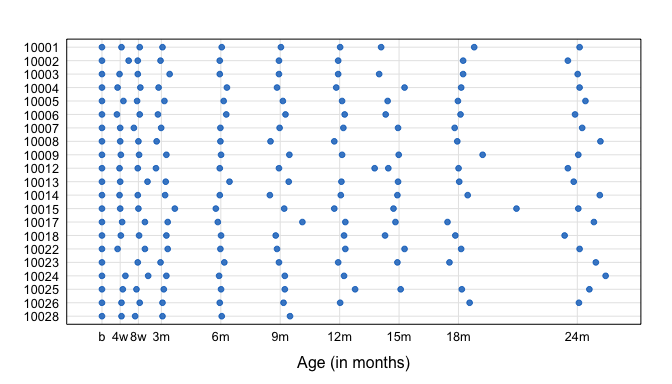Abacus plot of observation times for the first 20 children of the SMOCC data.

The blue points in Figure 1 indicate the observation times. In general, the blue points are close to the scheduled ages (indicated by vertical lines), especially in the first half year. Observation times vary more for older children. Several children have one or more missing visits (e.g. 10002, 10008, 10024). Some children (10012, 10015) had fairly close visits. Child 10028 dropped out after month 9.

Let us fit two models, with two and nine lines respectively, to the standard deviation score (SDS) of body length.

ids <- c(10001, 10005, 10022)
fit2 <- brokenstick(hgt.z ~ age | id, smocc_200, knots = 0:3)
knots <- c(0, 0.0833, 0.1667, 0.25, 0.5, 0.75, 1, 1.25, 1.5, 2)
fit9 <- brokenstick(hgt.z ~ age | id, smocc_200,
knots = knots, boundary = c(0, 3))
m2 <- plot(fit2, smocc_200, group = ids,
xlab = "Age (years)", ylab = "Length (SDS)")
m9 <- plot(fit9, smocc_200, group = ids,
xlab = "Age (years)", ylab = "Length (SDS)")
gridExtra::grid.arrange(m2, m9, nrow = 2)
## Warning in checkConv(attr(opt, "derivs"), opt$par, ctrl = control$checkConv, :
## Model failed to converge with max|grad| = 0.00772396 (tol = 0.002, component 1)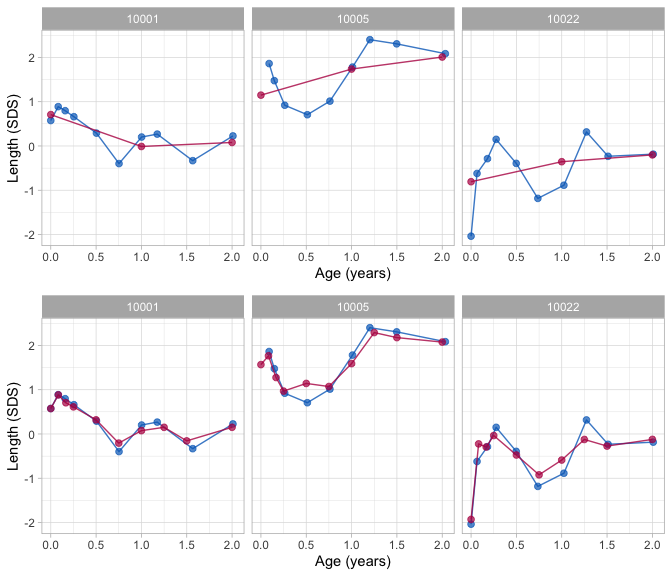Broken stick model with two (top) and nine (bottom) line segments for three children. Blue = observed data, Red = Fitted broken stick curves.

Figure 2 shows the individual trajectories of three children. The blue points coincide with the observed data, whereas the red curves are calculated according to the broken stick model.

There are fitted two models. The simpler model (top) uses just two line segments. The first line starts at birth and ends at the age of exactly 1 years. The second line spans the period between 1 to 2 years. Note that the two lines connect at the breakpoint, the age of 1 year. The red curves for the two-line model are a crude approximation to the data.

We can create a better model by setting breakpoints equal to the scheduled ages. Since there are 10 scheduled ages, we construct nine straight lines. In contrast to the two-line model, the nine-line broken stick model is sensitive to small bumps in the observed trajectory and closely fits the empirical data. The residual variance of the nine-line model is low (0.059), and the proportion of explained variance in SDS is high, 0.98.

While the observation times in the data differ between children, the broken stick curves use identical time points across subjects. The idea is now that we can add the broken stick estimates to the child-level data by a long-to-wide conversion, and analyse supplemented columns as repeated measures. A repeated measures analysis is usually simpler than the equivalent for the temporally misaligned data. For example, it is easy to calculate mean profiles for arbitrary groups, estimate the time-to-time covariance matrix or to build predictive models at the child level. See Hand and Taylor (1987) for a lucid overview of linear techniques for repeated measures.

# Methodology

## Notation

We adopt the notation of Fitzmaurice, Laird, and Ware (2011). Let $$Y_{ij}$$ denote the response variable for the $$i^{\rm th}$$ subject on the $$j^{\rm th}$$ measurement occasion at time $$t_{ij}$$. Data are collected in a sample of $$N$$ persons $$i=1,\dots,N$$. Let repeated measurements for the $$i^{\rm th}$$ subject be grouped as $Y_i = \left( \begin{array} {l} Y_{i1} \\ Y_{i2} \\ \vdots \\ Y_{in_i} \end{array} \right), \quad i = 1, \dots, N.$ If the measures have been observed at a common same set of occasions, then we could drop the index $$i$$ in $$t_{ij}$$ since $$t_{ij} = t_j$$ for all $$i = 1, \dots, N$$. Here we will focus on the case that $$t_{ij}$$ varies over $$i$$.

In addition, let use define the $$n_i \times p$$ matrices $X_i = \left( \begin{array} {llll} X_{i11} & X_{i12} & \cdots & X_{i1p} \\ X_{i21} & X_{i22} & \cdots & X_{i2p} \\ \vdots & \vdots & \ddots & \vdots \\ X_{in_i1} & X_{in_i2} & \cdots & X_{in_ip} \end{array} \right), \quad i = 1, \dots, N,$ so that the rows of $$X_i$$ contain $$p$$ covariates associated with the responses at $$n_i$$ measurement occasions. The columns may be time-varying covariates. If a certain covariate is fixed in time (e.g. sex, treatment, education), then all values within the corresponding column in $$X_i$$ are identical.

## Broken stick model

The broken stick model avoids modeling observation times $$t_{ij}$$ directly by representing each $$t_{ij}$$ as its relative position within a time interval. For example, suppose $$t_{ij} = 0.6$$ years and that the time interval is given by 0.5-1.0 years. The position relative to the left break age is $$x_{\rm left} = (1.0-0.6)/(1.0-0.5) = 0.8$$, whereas relative to the right break age is $$x_{\rm right} = (0.6-0.5)/(1.0-0.5) = 0.2$$. In order to fit the broken stick model, we need to replace time point $$t_{ij} = 0.6$$ by two values: 0.8 (for break age 0.5), and 0.2 (for break age 1.0). Note that both values add up to 1. Coding time in this way simplifies modeling continuous time by a set of discrete break ages.

More specifically, let $$t_{ij}$$ be coded by a second-order (linear) B-spline using $$k$$ internal knots $$\kappa$$ placed at $$k+1$$ ordered ages $\kappa_0 = \kappa_1 < \dots < \kappa_k < \kappa_{k+1}$ The internal knots $$\kappa_1, \dots, \kappa_k$$ correspond to the set of ages for which we obtain broken stick estimates, and it could be specified by the user. The left boundary knot $$\kappa_0 = \kappa_1$$ is left-anchored to the minimum time $$\min(t_{ij})$$ in the data. This point defines the starting event of the participant, such as birth or study enrolment. The right hand boundary knot is $$\kappa_{k+1} \geq \max(t_{ij})$$.

The second-order B-spline (de Boor 1978, 32), $H_s(t) = \left\{ \begin{array} {l@{\quad,\quad}l} (t-\kappa_{s-1})/(\kappa_s - \kappa_{s-1}) & \kappa_{s-1} < t \leq \kappa_s,\\ (\kappa_{s+1}-t)/(\kappa_{s+1} - \kappa_s) & \kappa_s \leq t < \kappa_{s+1},\\ 0 & {\rm otherwise.} \end{array} \right.$ is applied to $$t_{ij}$$ to obtain $$(k+1)$$ transformed variables $$x_{is} = t_{ij}$$ with $$s = 1,\dots,k+1$$. These variables can conveniently be grouped into the $$n_i \times (k+1)$$ matrix of covariates $$X_i = (x_{i1}, \dots, x_{ik}, x_{i(k+1)})$$. Each row in $$X_i$$ has only one or two non-zero elements, which sum to 1.

Using this $$X_i$$, the broken stick model is a special case (with $$Z_i = X_i$$) of the two-stage random-effects model (Laird and Ware 1982)

$Y_i = X_i\beta + X_ib_i + \epsilon_i$

where the $$k+1$$ column vector $$\beta$$ contains $$k+1$$ fixed effect coefficients common to all persons, where the $$k+1$$ column vector $$b_i$$ accomodates for $$k+1$$ subject-specific random parameters, and where the $$n_i$$ column vector $$\epsilon_i$$ holds subject-specific residuals.

In order to complete the model specification, we assume that the residuals are identically and independently distributed as $$\epsilon_i \sim N(0,\sigma^2 I(n_i))$$, where $$\sigma^2$$ is a common variance parameter, and where $$I(n_i)$$ is the identity matrix of order $$n_i$$. Thus, the equation represents population parameters (fixed effects), individual effects (random effects), and an amount of within-person dispersion that is the same for all persons. The section on estimation also considers a heterogeneous model that allows $$\sigma_i^2$$ to vary over subjects.

In summary, given the knot specification and the choice of the response scale, the parameters of the broken stick model are:

• $$\beta$$, a vector of $$k + 1$$ fixed parameters;
• $$\Omega$$, a $$(k+1) \times (k+1)$$ covariance matrix of the random effects;
• $$\sigma^2$$, the within-person error variance.

The total number of parameters for a solution with $$k$$ internal knots is thus equal to $$(k^2 + 5k + 6)/2$$. For example, a model of $$k = 3$$ knots (i.e. with two connected lines) has 15 parameters, a model with $$k = 4$$ has 21 parameters, and a model with $$k = 10$$ break ages has 78 parameters.

## Model assumptions

At the person level, we assume $$b_i \sim N(0, \Omega)$$, i.e., the random coefficients of the subjects have a multivariate normal distribution with zero mean and a $$(k+1) \times (k+1)$$ covariance matrix $$\Omega$$. The base model allows the elements of $$\Omega$$ to vary freely. For time-dependent data, constrained versions for $$\Omega$$ are also of interest.(Fitzmaurice, Laird, and Ware 2011, ch. 7). The estimation section highlights two such extensions. We also assume that the covariance between $$b_i$$ and $$\epsilon_i$$ is zero. For simplicity, this paper is restricted to the case where $$X_i$$ includes only time, and no other covariates. Also, we assume that $$X_i$$ has no missing data.

The broken stick model builds upon three main modeling assumptions:

• The trajectory between break ages follows a straight line. This assumption may fail for processes that are convex or concave in time. For example, human height growth in centimeters growth is concave, so setting breakpoints far apart results introduces systematic model bias. Modeling height SDS instead of raw height will prevent this bias.
• The broken stick estimates follow a joint multivariate normal distribution. As this assumption may fail for skewed measurements, it could be beneficial to transform the outcomes so that their distribution will be closer to normal.
• The data are Missing at Random (MAR) given the outcomes from all subjects at all observation times. This assumption is restrictive in the sense that missingness may only depend on the observed outcomes, and not on covariates other than time. At the same time, the assumption is liberal in the sense that the missingness may depend on future outcomes. While this MAR-future assumption is unusual in the literature on drop-out and observation time models, it is a sensible strategy for creating imputations that preserve relations over time, especially for intermittent missing data. Of course, the subsequent substantive analysis on the imputed data needs to be aware of the causal direction of time.

## Interpretation

Given the model estimates and the person data, we can calculate the random effect $$b_i$$. The broken stick parameter $$\gamma_{is} = \beta_s + b_{is}$$ is the subject-specific mean of $$Y_i$$ at time $$\kappa_s$$, $$s = 1,\dots, k + 1$$. The set of $$\gamma_{is}$$ parameters describes the mean response profile for subject $$i$$ by $$k$$ lines that connect at the $$k + 1$$ coordinates $$(\kappa_s, \gamma_{is})$$.

The broken stick parameter is the most likely value of outcome $$Y_i$$ for subject $$i$$ at time $$\kappa_s$$. The parameter is the centre of the posterior predictive distribution for normal $$Y_i$$. The two-sided $$100(1-\alpha)\%$$ prediction interval for the true, though often unobserved, value $$Y_{i,\kappa_s}$$ is equal to

$[Y_{i,\kappa_s}^{\rm lo}, Y_{i,\kappa_s}^{\rm hi}] = \gamma_{is} \pm t_{(1-\alpha/2;N-1)}\sigma,$ where $$t_{(1-\alpha/2;N-1)}$$ is the $$100(1-\alpha/2)$$ percentile of Student’s $$t$$-distribution with $$N - 1$$ degrees of freedom. For example, the 50% prediction interval $$\gamma_{is} \pm 0.68\sigma$$ will contain 50% of true values. For normal $$Y_i$$, the length of the 50% prediction interval is equivalent to the interquartile range (IQR). If the residual variation $$\sigma^2$$ is small (say $$\sigma^2 < 0.1$$), the IOR is about 0.22, so half of the true values will be within 0.22 SD of $$\gamma_{is}$$, a small difference. For large $$\sigma^2$$ (e.g. $$\sigma^2 > 0.2$$), the $$\gamma_{i}$$ vector is a smoothed representation of $$Y_{i}$$. While smoothness amplifies low-frequency features of the trajectories, it could also introduce biases in the subsequent analysis by suppressing high-frequency variation. In that case, the analyst needs to check whether this reduction in variation does not affect the parameters of substantive interest. We may restore high-frequency variation by adding random draws from the residual distribution $$N(0, \sigma^2)$$. From there, it is a small step to multiple imputation, a well-developed methodology for drawing valid inferences from incomplete data.(Rubin 1987; van Buuren 2018)

If $$n_i >> k$$ then the broken stick model provides a parsimonious representation of the measurements. Reversely, if $$n_i << k$$ then the model infers plausible values for subject $$i$$ by building strength across persons. The broken stick model converts $$n_i$$ irregularly observed measurements into a new set of $$k$$ values $$\gamma_{is}$$ at common ages $$\kappa_1, ..., \kappa_k$$, $$s = 1,\dots, k$$.

Since each row in $$X_i$$ sums to unity, the broken stick model does not have a global intercept. The linear B-spline coding effectively replaces the global random intercept term by $$k + 1$$ local intercepts, one at each break age. The local intercept summarizes the information available in the adjacent left and right age intervals and ignores any information beyond the two adjacent knots. The broken stick estimates are thus primarily local. Outcome data observed outside the two adjacent age intervals influence the broken stick estimates only through the subject-level part of the model, in particular through $$\Omega$$.

## Estimation

### Parameter estimation, method lmer

Estimation of the broken stick model relies on two well-developed functions: splines::bs() (R Core Team 2020) and lme4::lmer().(Bates et al. 2015) The following snippet illustrates how the brokenstick::make.basis() function calculates the matrix of $$B$$-splines for the time variable age:

library(splines)
data <- brokenstick::smocc_200
brk <- c(0, 0.5, 1, 2)
X <- bs(data$age, knots = brk, Boundary.knots = c(0, 3), degree = 1) colnames(X) <- paste("age", c(brk, 3), sep = "_") data <- cbind(data[, c("id", "age", "hgt.z")], X) head(data) ## id age hgt.z age_0 age_0.5 age_1 age_2 age_3 ## 1 10001 0.000 0.57 1.00 0.00 0.0000 0 0 ## 2 10001 0.082 0.89 0.84 0.16 0.0000 0 0 ## 3 10001 0.159 0.80 0.68 0.32 0.0000 0 0 ## 4 10001 0.255 0.66 0.49 0.51 0.0000 0 0 ## 5 10001 0.504 0.29 0.00 0.99 0.0076 0 0 ## 6 10001 0.753 -0.40 0.00 0.49 0.5058 0 0 The numerical example shows that the bs() function transforms the age variable into five columns, the $$B$$-spline basis, with names like age_0 and age_0.5. If age coincides with one of these (e.g., as in the top row), then the corresponding column receives a 1. In all other cases, age distributes over two adjacent columns. To make things fit, we need an additional column (here age_3) at the last position, the right boundary knot. There is also a left boundary knot, and I have conveniently set that equal to the first breakpoint, marking the start of time. Setting degree = 1 specifies a $$B$$-spline gives the broken stick model its name and its characteristic shape. To illustrate the second step of the calculations, we call lme4::lmer() as follows: library(lme4) ctl <- .makeCC("warning", tol = 4e-3) f <- hgt.z ~ 0 + age_0 + age_0.5 + age_1 + age_2 + age_3 + (0 + age_0 + age_0.5 + age_1 + age_2 + age_3 | id) fit <- lmer(f, data, control = lmerControl(check.conv.grad = ctl)) ## Warning in checkConv(attr(opt, "derivs"), opt$par, ctrl = control$checkConv, : ## Model failed to converge with max|grad| = 0.0066811 (tol = 0.004, component 1) ### fitted trajectories for all persons bse <- t(t(ranef(fit)$id) + fixef(fit))
head(round(bse, 3), 3)
##       age_0 age_0.5  age_1  age_2  age_3
## 10001  0.77    0.26 -0.005  0.088 -0.148
## 10002 -0.27   -0.21 -0.465 -0.479 -0.052
## 10003  1.65    1.96  1.294  1.129 -1.323

The broken stick estimates are the sum of the fixed and random effects. We need to remove the intercept as predictor rows all sum to 1. Warnings often occur when fitting broken stick models with lme4::lmer(). Here we have surpressed the warning ## Warning in checkConv(attr(opt, "derivs"), opt$par, ctrl = control$checkConv, : Model failed to converge with max|grad| = 0.0031397 (tol = 0.002, component 1) by setting tol to a more relaxed value. I have found that the broken estimates still look sound and reasonable. Most of my experience derives for child growth data, so there is no guarantee that this apparent robustness will hold for other types of data. Warnings become less frequent for a lower number of breakpoints and larger samples sizes.

The package can fit the same model in an easier way:

ctl <- control_brokenstick(
.makeCC("warning", tol = 4e-3)))
mod <- brokenstick(hgt.z ~ age | id, data = smocc_200,
knots = c(0, 0.5, 1, 2), boundary = c(0, 3),
control = ctl)
## Warning in checkConv(attr(opt, "derivs"), opt$par, ctrl = control$checkConv, :
## Model failed to converge with max|grad| = 0.0066811 (tol = 0.004, component 1)
head(predict(mod, smocc_200, x = "knots", shape = "wide"), 3)
## # A tibble: 3 x 6
##      id    0  0.5      1     2     3
##   <dbl>  <dbl>  <dbl>    <dbl>   <dbl>   <dbl>
## 1 10001  0.772  0.259 -0.00513  0.0883 -0.148
## 2 10002 -0.269 -0.206 -0.465   -0.479  -0.0524
## 3 10003  1.65   1.96   1.29     1.13   -1.32

### Parameter estimation, method kr

The calculation time of lme4::lmer() rapidly increases with the number of random effects. More than ten random effects (knots) takes significant time, and beyond 15 knots is generally impossible to fit. The package provides another alternative, the Kasim-Raudenbush (KR) sampler (Kasim and Raudenbush 1998), which simulates draws from the posterior distributions of parameters from a two-level normal model with heterogeneous within-subject variances. The speed of the KR-Raudenbush sampler is almost insensitive to the number of random effects and depends primarily on the total number of iterations. The brokenstick::kr() function provides some reasonable defaults. The behaviour of the method has not been studied as well as lmer() and should still be considered experimental.

Apart from being faster, the KR-sampler opens up interesting analytic options:

1. It is relatively easy to constrain the fitted covariance of random effects, $$\Omega$$, to a matrix of simple structure. Informing the sampler of the time-dependent structure of the random effect leads to stabler estimates of $$\Omega$$. The package currently implements two correlations models. These models express the correlation $$\rho(t_1, t_2)$$ between two Z -scores $$Z_1$$ and $$Z_2$$ at successive ages $$t_1$$ and $$t_2$$ as a function of those ages. The Argyle model (Argyle, Seheult, and Wooff 2008) is $$\rho(t_1, t_2) = \exp(-\lambda|T_1-T_2|)$$, where $$T_i = \log(\tau+t_i)$$ is a logarithmic rescaling of the time axis and $$\rho=exp(-\lambda)$$. The Cole correlation model (Cole 1995) describes the Fisher-transformed correlation as a function of the average $$(t_1+t_2)/2$$ and the difference $$(t_2-t_1)$$, including two multiplicative terms. Note that both models were proposed in the context of child growth, and have not been tested for other types of time-dependent data.

2. The KR-sampler fits the slightly more general linear-mixed model with heterogeneous within-subject variances, i.e. with a residual variance $$\sigma_i^2$$ per subject $$i$$ instead of the global residual $$\sigma^2$$. This makes it easier to identify, study and weight subjects based on how well they fit the model.

3. A third option is to simulate imputations as an extra step to the KR-sampler. For subject with large $$\sigma_i^2$$, the random effect estimates are a too smooth representation of the data, leading to inappropriate variance estimates when those estimates are analysed as “just data”. Section 11.3 of van Buuren (2018) pioneered a solution that constructs multiple trajectories by adding a proper amount of residual noise to random effect estimates. The variance estimation then proceeds according to the principles of multiple imputation.(Rubin 1987)

### Random effects estimation

Apart from parameter estimates of the broken stick model, we also need a way to estimate random effects for a given set of model estimates and (new) user data. There are several of such methods. The brokenstick::EB() function implements the empirical Bayes (EB) estimate, also known as BLUP (Skrondal and Rabe‐Hesketh (2009), p. 683). The procedure can provide the broken stick estimates for new persons. It the workhorse of the more user-friendly predict() method that takes fitted models objects.

# Functionality

## Overview of brokenstick package

The package contains functions to fit, predict and plot data. The main functions in the brokenstick package are:

Function name Description
brokenstick() Fit a broken stick model to irregular data
predict() Predict broken stick estimates for new data
plot() Plot individual trajectories

The following functions are user-oriented helpers:

Function name Description
fitted() Calculate fitted values
get_knots() Obtain the knots used by model
get_r2() Obtain proportion of explained variance
residuals() Extract residuals from model

The following functions are responsible for calculations:

Function name Description
control_brokenstick() Set controls to steer calculations
EB() Empirical Bayes predictor for random effects
kr() Kasim-Raudenbush sampler for two-level model
make_basis() Create linear splines basis

The package follows the conventions. For example, the modelling object does not store the training data, whereas the convention dictates the variable names. The package architecture borrows important ideas from the package.(Vaughan and Kuhn 2020)

## Data preparation

Before we can fit the model, the data need to be in shape. Data preparation is often the most time-consuming part of the analysis. The brokenstick() function takes tidy data in the long-form, with every observed subject-time combination in a row. This section uses the built-in smocc_200 data, containing the heights of 200 children measured at ten visits up to two years.(Herngreen et al. 1994)

library(brokenstick)
head(smocc_200, 3)
## # A tibble: 3 x 7
##      id    age sex       ga    bw   hgt hgt.z
##   <dbl>  <dbl> <chr>  <dbl> <dbl> <dbl> <dbl>
## 1 10001 0      female    40  3960  52   0.575
## 2 10001 0.0821 female    40  3960  55.6 0.888
## 3 10001 0.159  female    40  3960  58.2 0.797

## Calculate Z-scores

The broken stick model can fit observations in either the raw scale (cm, kg, and so on) or as a standard deviation score (SDS), or $$Z$$-score. The results from the analysis of the $$Z$$-score is preferable for several reasons:

1. for growth curves, a straight line assumption is more plausible in the $$Z$$-score scale;
2. observations in the $$Z$$-score scale are closer to multivariate normality;
3. analysis of $$Z$$-scores highlights the interesting variation within and between children;
4. fitting $$Z$$-score data leads to fewer convergence issues.

It is easy to convert the measurements into the $$Z$$-score scale, fit the model, and convert back to the raw scale afterwards, if desired. There are several packages that assist in the calculations: , , , and .

The smocc_200 data contains the height measurement both in the original scale in cm (hgt) and the $$Z$$-score scale (hgt.z) relative to the height references from the Fourth Dutch Growth study (Fredriks et al. 2000). Let us recalculate height SDS using the package. Fortunately, we find that the same values.

library(AGD)
z <- with(smocc_200, y2z(y = hgt,
x = age,
sex = ifelse(sex == "male", "M", "F"),
ref = nl4.hgt))
identical(z, smocc_200$hgt.z) ##  FALSE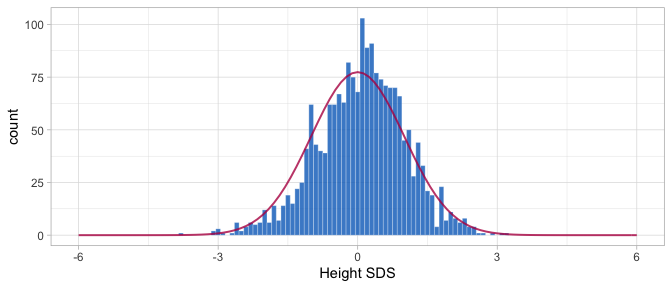Distribution of height SDS for 200 Dutch children. Figure 3 shows that, as expected, the empirical Z-score distribution is close to the standard normal. The few very extremely low heights correspond to pre-term born infants. The next section concentrate on modelling hgt.z. Function z2y() applies the inverse transformation of $$Z$$-scores to the original scale. The following snippet converts hgt.z into the cm scale. y <- with(smocc_200, z2y(z = hgt.z, x = age, sex = ifelse(sex == "male", "M", "F"), ref = nl4.hgt)) all.equal(y, smocc_200$hgt, tol = 0.0001)
##  "Mean relative difference: 0.003"

We have used the Dutch 1997 height references here, but there are more choices. The package also supports the WHO Child Growth Standards.

In practice we found that the model fit is often better when applied to $$Z$$-scores. Age-conditional references are common in child growth exists, but could be rare in other fields. An alternative is to apply the broken stick model to the standardized residuals of a preliminary non-linear regression of the outcome on time.

## Model fitting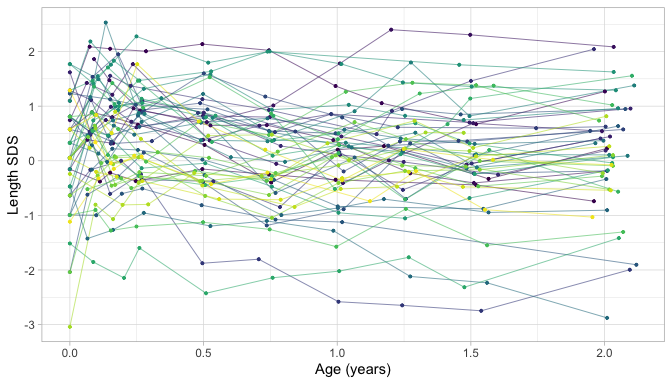Length growth of 52 infants expressed in the Z-score scale.

Figure 4 displays the growth curves of a subset of 52 children. The $$Z$$-score transformation takes away the major time trend, so all trajectories are more or less flat. This display allows us to see an extremely detailed assessment of individual growth. Note how the measurements cluster around ten ages: birth, 1, 2, 3, 6, 9, 12, 15, 18 and 24 months. While the data collectors rigorously followed the study design, variation in timing is inevitable because of weekends, holidays, sickness, and other events.

### Fit one line

As a start, let us fit a simple model with just one line anchored at the minimum and maximum age.

fit <- brokenstick(hgt.z ~ age | id, smocc_200)
ids <- c(10001, 10005, 10022)
plot(fit, new_data = data, group = ids, what = "all",
xlab = "Age (years)", ylab = "Length (cm)")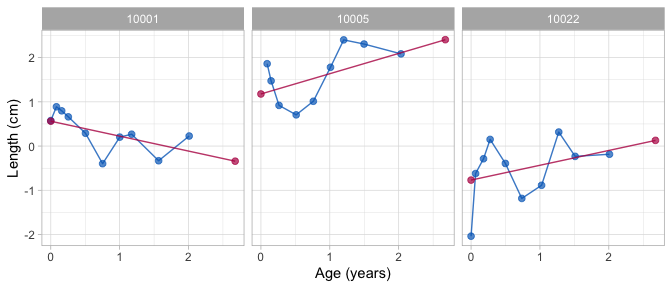Simple linear model with one line anchored at the extremes.

Figure 5 shows the observed (blue) and fitted (red) trajectories of three selected children. Note that this model can only capture the overall age trend. As a result, the approximation to the data is quite bad.

### Fit two lines

We now extend to two connected lines. The first line should start at birth and end at the age of one year. The second line spans the period between one to two years. The lines must connect at the age of one year. We estimate and plot the model as follows:

fit2 <- brokenstick(hgt.z ~ age | id, smocc_200, knots = 0:2)
plot(fit2, data, group = ids,
xlab = "Age (years)", ylab = "Length (SDS)")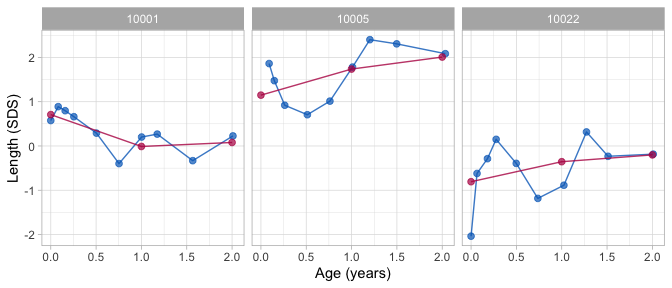Broken stick model with two lines.

The fit2 object holds the parameter estimates of the model:

fit2
## Class: brokenstick (lmer)
## Knots: 0 1 2 2.7
## Means: 0.09 0.04 0.09 0.12
## Variance-covariance matrix:
##            age_0 age_1 age_2 age_2.6776
## age_0       0.83
## age_1       0.42   0.8
## age_2       0.44  0.76  0.83
## age_2.6776 -0.19  0.12  0.23       0.29
## Residual variance:  0.18

The printed output lists the knots of the model at 0, 1, 2 and 2.6776 years. The left and right boundaries are located at 0 and 2.6776, respectively. The means entry lists the fixed effect estimates, which we interpret as the average SDS per time point. The time-to-time variance-covariance matrix cover four random effects (3 visits + 1 end knot). The residual variance measures the variability of the discrepancies between the model and the observed data. These three parameters (fixed, random, residual variance) are well interpretable and fully record the fitted broken stick model.

### Fit nine lines

The two-line model does not fit well. We substantially refine the model by adding a knot for each scheduled visit. To make model specification independent of the data, we specify the right boundary as a constant of three years. We code and run the model as

knots <- round(c(0, 1, 2, 3, 6, 9, 12, 15, 18, 24)/12, 4)
fit9 <- brokenstick(hgt.z ~ age | id, data = smocc_200,
knots = knots, boundary = c(0, 3))

This optimization problem is more complicated and time-consuming. As noted before, it is common for the optimization software to issue warnings, often related to the number of random effects relative to the number of observations. While these may be a little discomforting, we have found that the warnings are generally at the conservative side, and that the parameter estimates seem OK. With a small residual variance of 0.059, the nine-line broken stick model fits the observed data very well.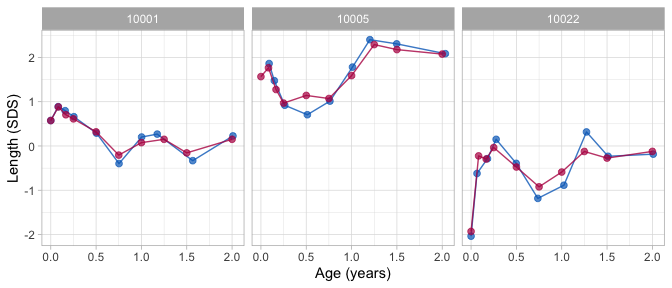Broken stick model with nine lines.

The training set includes all subjects. Depending on the study goals, we may wish to further improve the model fit by removing children from the data. For example, there might be children for which few observations are available, children with diseases, or children with trajectories that are very unusual or faulty. Be aware that such removals preserve the external generalisability of the training sample.

## Prediction

Once we have a fitted model, we may obtain predictions. The subject(s) could be part of the training sample, but could also consist of new children.

### All subjects

The predict() function obtains predictions from the broken stick model. The function is flexible, and allows for prediction of new subjects at arbitrary ages in a variety of output formats. The simplest call

p1 <- predict(fit9, smocc_200)
head(p1, 3)
##   .pred
## 1  0.57
## 2  0.88
## 3  0.72

produces the predicted value (in .pred) for each row in data.

The predicted values represent a compromise between the person’s data values and the global mean. In general, the fewer and less extreme data points of a person are, the closer the compromise will be toward the global mean. The compromise is called the conditional mean of the posterior distribution, the sum of the fixed and random effects.

We can obtain the locations at which the lines connect by specifying the x = "knots" argument, e.g.

p2 <- predict(fit9, smocc_200, x = "knots")
head(p2, 3)
## # A tibble: 3 x 9
##   .source    id    age sex      ga    bw   hgt hgt.z .pred
##   <chr>   <dbl>  <dbl> <chr> <dbl> <dbl> <dbl> <dbl> <dbl>
## 1 added   10001 0      <NA>     NA    NA    NA    NA 0.574
## 2 added   10001 0.0833 <NA>     NA    NA    NA    NA 0.883
## 3 added   10001 0.167  <NA>     NA    NA    NA    NA 0.705

This is case 1 in the help of predict.brokenstick(). The result p2 is a table with 2200 rows (= 11 knots $$\times$$ 200 subjects). The rows include additional identifying information. Adding the shape = "wide" argument transforms the information into repeated measures, with 200 rows and 12 = 1 + 11 columns, that form supplemental variables for further analyses at the subject level.

We may also obtain both the conditional means as well as predictions at the observation ages for all children by

p3 <- predict(fit9, smocc_200, x = "knots", strip_data = FALSE)
head(p3, 3)
## # A tibble: 3 x 9
##   .source    id    age sex       ga    bw   hgt hgt.z .pred
##   <chr>   <dbl>  <dbl> <chr>  <dbl> <dbl> <dbl> <dbl> <dbl>
## 1 data    10001 0      female    40  3960  52   0.575 0.574
## 2 data    10001 0.0821 female    40  3960  55.6 0.888 0.878
## 3 data    10001 0.159  female    40  3960  58.2 0.797 0.721

which contains 4140 rows (= 1940 data points + 2200 added points).

Now suppose that we desire to predict height SDS at other ages, e.g. at 0.42, 1.33 and 4 years. We can do so by (case 4, all groups)

head(predict(fit9, smocc_200, x = c(0.42, 1.33, 4), shape = "wide"), 3)
## # A tibble: 3 x 4
##      id 0.42  1.33   4
##   <dbl>  <dbl>   <dbl> <dbl>
## 1 10001  0.413  0.0524    NA
## 2 10002 -0.261 -0.477     NA
## 3 10003  2.06   1.06      NA

Thus, we have some flexibility to work with times that are not breakpoints. Remember though that the underlying model did not change. For example, we cannot magically predict outside the model at age 4.

### Single subject

Obtaining predicted values per subject requires the group argument (case 3). For example

predict(fit9, smocc_200, group = 10001, shape = "vector")
##    0.574  0.878  0.721  0.607  0.312 -0.203  0.076  0.128 -0.115  0.155

returns the vector of predictions for child 10001. Remove the shape argument to append the child’s data. Also, here we can predict at other times using the x argument (case 4).

Now suppose that for subject 10001 we have additional height data at ages 0.42 and 1.33 years. Can we predict the child’s trajectory with these new points included? The answer is yes. The command (case 5)

tail(predict(fit9, smocc_200, x = c(0.42, 1.33), y = c(-0.5, -1),
group = c(10001, 10001), strip_data = FALSE), 3)
## # A tibble: 3 x 9
##   .source    id   age sex       ga    bw   hgt  hgt.z   .pred
##   <chr>   <dbl> <dbl> <chr>  <dbl> <dbl> <dbl>  <dbl>   <dbl>
## 1 data    10001  2.01 female    40  3960  88.3  0.227  0.0765
## 2 added   10001  0.42 <NA>      NA    NA  NA   -0.5    0.230
## 3 added   10001  1.33 <NA>      NA    NA  NA   -1     -0.244

appends two new records to the data of child 10001, and recalculates the trajectory using all data.

### New subject

Suppose we have measured two children, Fred and Alice. We wish to obtain predictions for both using the model fit9. The following snippet calculates predictions at both the observed ages and at the knot locations:

data <- data.frame(
age = c(0, 0.12, 0.32, 0.62, 1.1, 0.25, 0.46),
hgt.z = c(-1.2, -1.8, -1.7, -1.9, -2.1, -1.9, -1.5),
id = c(rep("Fred", 5), rep("Alice", 2)))
p <- predict(fit9, data, x = "knots", strip_data = FALSE)

We can plot the trajectories data by

plot(fit9, data, ylim = c(-2.5, 0), xlab = "Age (years)", ylab = "Length (SDS)")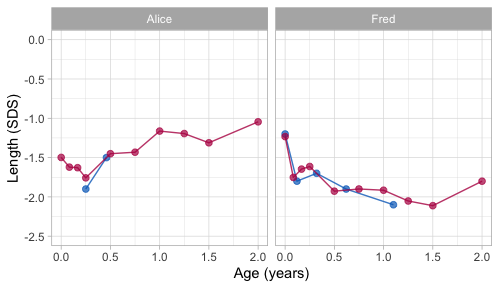Alice and Fred - observed (blue) and fitted (red) trajectory.

Alice contributes only two data points in the first half-year. The model expects that her height SDS will be around -1 SD at the age of two years. Using the data up to 1.1 years, the model predicts that Fred’s growth curve remains around -2.0 SD until Fred is 1.5 years, and then increases to around -1.8 SD. While both predicted trajectories are extreme extrapolations, the example illustrates that it is possible to make informed predictions using just a handful of data points.

The predict() function does not care about whether new_data is the training data or not. All options are supported in both training and test data, thus providing ample flexibility to suit many use cases.

## Quality of prediction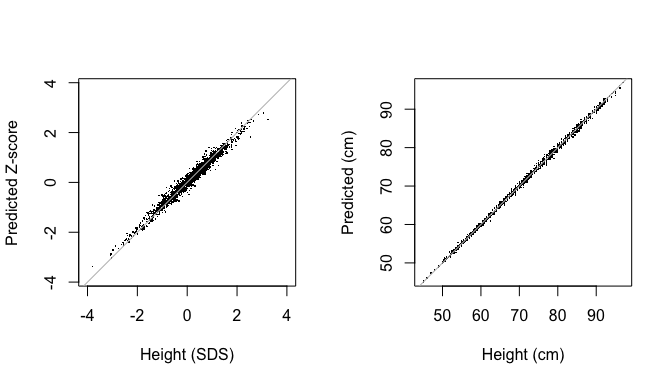Predicted versus observed values.

Figure 9 is the scatterplot of the observed versus predicted values provides a visual representation of the accuracy of the prediction of the model in height SDS and cm scales. Both plots suggest an excellent fit between the observed and fitted data. The percentage of explained variance for the height SDS is high: 97.8%. The standard deviation of the residuals is equal to 0.152 SD, a small value in the $$Z$$-scale. When back-converted to centimetres, the scatterplot of the observed versus predicted values is even a little tighter. The proportion of explained variance is close to perfection: 99.9%. The standard deviation of the residuals is 4 mm, about the size of the technical error of measurement (TEM) for duplicate measurements in infants.(Ismail et al. 2016, Table 2)

The model is as good as it can get. The uncertainties associated with the transformation from varying observation times to repeated measures will be small. For all practical purposes, the results from a linear mixed or multilevel model and a repeated measures model are likely to be same.

## Knot placement strategies

Fitting the broken stick model requires a specification of the knots. The choice of the knots influences the quality and usefulness of the solution, so exercise some care in setting appropriate knot locations.

The brokenstick() function uses the same set of knots for all subjects. By default, the procedure places the boundary knots at the range of the data and no inner knots, resulting in a model that is linear in time without breakpoints. The k argument is a quick way to add k internal knots at equidense quantiles of the time variable. For example, specifying k = 1 puts a knot at the 50th quantile (median), setting k = 3 puts knots at the 25th, 50th and 75th quantiles, and so on. While convenient and quick, this option can result in suboptimal knot placement that is not adequate for the problem at hand. In general, it is best to specify explicit values for the knots and boundary arguments.

Here are some suggestions for knot placement:

1. If you want to predict at specific ages, then specify knots at those ages. For example, if the scientific interest includes prediction at the age of 1 and 2 years, then include these ages as knots;
2. Setting knots at scheduled visits is a sensible strategy for obtaining predictions at precisely the scheduled times. Set equidistant knots if the analysis requires a fixed time interval;
3. Keep the number of knots low, for speed and simplicity. Having many ($$\geq 10$$) knots can improve the fit to the data. Still, it will also increase calculation time and may result in unstable solutions. For problems that require more than 10 knots, reduce calculation time by the setting method = "kr" method, and use control_kr() to improve stability by a correlation model;
4. Do not place knots in sparsely filled areas of the data, e.g. in-between two visits. Doing so may result in erratic joins;
5. Define a starting time common to all subjects (e.g. birth) and set the first breakpoint knots equal to the left boundary knot boundary. The brokenstick() is already cautions to ensure this;
6. Order knots in size;
7. Use the get_knots() function to extract knots from a fitted model;
8. Set maximum value in knots to the highest time of scientific interest, but still within the data range. Set boundary knot boundary larger than this value, e.g. equal to the maximum of the time variable. Broken stick estimates at the right boundary knot have no useful interpretation, so exclude those estimates from plots and ignore them in subsequent analyses;
9. Rule of thumb: Limit the number of knots to the (average) number of data points per subject;
10. Set knots to explicit values to support generalisation over the time variable in the training data.

# Applications

## Critical periods

The following question motivated the development of the broken stick model: At what ages do children become overweight? Knowing the answer to this question provides handles for preventive interventions to counter obesity. Dietz (1994) suggested the existence of three critical periods for obesity at adult age: the prenatal period, the period of adiposity rebound (roughly around the age of 5-6 years), and adolescence. Obesity formed in these periods is likely to increase the obesity risk at adult age and its complications.

A growth period, bounded by ages $$T_1$$ and $$T_2$$, is critical for adult overweight if the following criteria hold: (de Kroon et al. 2010)

1. there is a significant difference in mean gain score $$Z_2-Z_1$$ between subjects with and without adult overweight;
2. the gain score $$Z_2-Z_1$$ has an independent contribution over $$Z_2$$ to the prediction of $$Z_{\rm adult}$$. It not only matters where you were at $$T_2$$ but also how you got there;
3. $$Z_2$$ correlates highly with $$Z_{\rm adult}$$, so it is easier (i.e. with higher sensitivity and specificity) to identify children at risk for adult overweight.

de Kroon et al. (2010) found that the age interval 2-6 years met all criteria for a critical period. Our re-analysis tests the requirements for the following age intervals: birth-4 months, 4 months-1 year, 1-2 years, 2-4 years, 4-6 years, 6-10 years and 10-14 years. Hence, we define the following break ages:

knots <- round(c(0, 1/3, 1, 2, 4, 6, 10, 14, 24, 29), 3)
labels <- c("birth", "4m", "1y", "2y", "4y", "6y", "10y", "14y", "24y", "")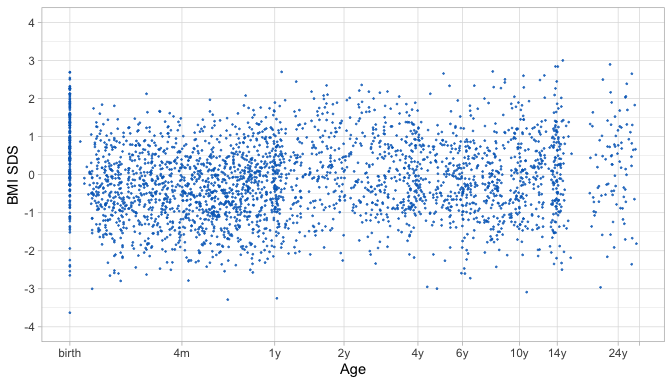Body Mass Index (BMI) SDS by log(age + 0.2) (Terneuzen cohort)

The Terneuzen Birth Cohort (de Kroon et al. 2008) comprises of 2604 children born around the year 1980 in Terneuzen, The Netherlands. Figure 10 shows the BMI standard deviation scores (SDS) against age in a random subset of 306 children. While we may easily recognise scheduled visits at birth, 1y and 14y, observations at other periods are less structured. Compared to the analysis in de Kroon et al. (2010), we removed the knots at 8 days and 18 years (because these appear in sparse data areas) and added knots at 4, 14 and 24 years. We set the right boundary knot to 29y, slightly higher than the maximum age in the data.

ctl <- control_brokenstick(
lmer = lmerControl(check.conv.grad = .makeCC("warning", 0.02, NULL),
check.conv.singular = .makeCC("ignore", 0.001)))
fit_lmer <- brokenstick(bmi.z ~ age | id, data = mice::tbc,
knots = knots, boundary = c(0, 29),
control = ctl)

Depending on the precise specification of the knots, the default brokenstick() procedure that calls lme4::lmer() may print the warning Model failed to converge with max|grad| = 0.011882 (tol = 0.002, component 1) or a message boundary (singular) fit: see ?isSingular. These issues arise because of the over-parametrised nature of the default broken stick model, potentially resulting in a singular variance-covariance matrix fit@omega, combined with a sparsity of data. The control_brokenstick() command can prevent the warning and message.

ids <- c(8, 1259, 2447, 7019, 7460, 7646)
plot(fit_lmer, mice::tbc, group = ids,
ylab = "BMI SDS", xlab = "Age (years)")